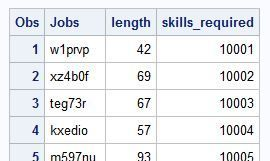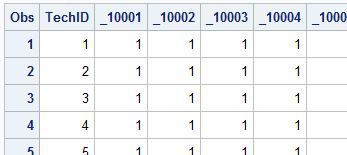## Constraint setup for skills/abiities/assignment in bin packing problem optmodel

Hi,

I am setting up an optmodel where jobs have to be assigned to technicians, but only those, who have the ability/skill required. This is similar to a job shop scheduling model where some tasks can only be executed by certain machines.

My input data consists of two arrays, a list of jobs array that contains all jobs and required skill per job (1) and a tech_skills array that contains the skills a technician has (2). The format is as follows:

Job list array  (PRODUCTS):technician_skills array (SKILLS):My data is stored as follows:

proc optmodel;

/* read the product and size data */

set <str> PRODUCTS;

num length {PRODUCTS};

num skills_required {PRODUCTS};

read data myContent into PRODUCTS=[Jobs] length skills_required;

/* read the tech and skills data */

set <num> SKILLS;

num _10001 {SKILLS};

num _10002 {SKILLS};

num _10003 {SKILLS};

num _10004 {SKILLS};

num _10005 {SKILLS};

num _10006 {SKILLS};

num _10007 {SKILLS};

num _10008 {SKILLS};

num _10009 {SKILLS};

num _10010 {SKILLS};

read data mytech_skills into SKILLS=[TechID] _10001 _10002 _10003 _10004 _10005 _10006 _10007 _10008 _10009 _10010;

I would like to set up a constraint that only allows the allocation of a job to a bin/machine/technician if the skill requirement is met. I imagine it should have the following structure:

for all j in JOBS:

for all s in SKILLS:

Tech_skills[ s ][ skills_required[j] ] * decision variable = 1;

The decision variable determines whether a job is executed by a technician or not (0,1).

I tried a couple or variants, but I think I do not access the variables correctly:

con SkillsCon {p in PRODUCTS}:

sum{b in BINS} SKILLS[b, skills_required[p]] * Assign[p,b] = 1;

Please let me know if any information is missing.

1 ACCEPTED SOLUTION

Accepted Solutions

## Re: Constraint setup for skills/abiities/assignment in bin packing problem optmodel

One way is to use a logical condition in the sum:

``````   con Capacity {t in TECHS}:
sum {j in JOBS: has_skill[t,skills_required[j]] = 1} length[j] * Assign[j,t] <= binsize * UseBin[t];
``````

Another way is to introduce JOBS_t like TECHS_j:

``````   set JOBS_t {t in TECHS} = {j in JOBS: has_skill[t,skills_required[j]] = 1};
con Capacity {t in TECHS}:
sum {j in JOBS_t[t]} length[j] * Assign[j,t] <= binsize * UseBin[t];
``````
7 REPLIES 7

## Re: Constraint setup for skills/abiities/assignment in bin packing problem optmodel

Assuming each job has only one required skill, here's one way to do it, with a two-dimensional array has_skill:

``````   set <str> JOBS;
num length {JOBS};
num skills_required {JOBS};
read data myContent into JOBS=[Jobs] length skills_required;
set SKILLS = setof {j in JOBS} skills_required[j];
set <num> TECHS;
num has_skill {TECHS, SKILLS};
read data mytech_skills into TECHS=[TechID] {s in SKILLS} <has_skill[TechID,s]=col('_'||s)>;
print has_skill;
set TECHS_j {j in JOBS} = {t in TECHS: has_skill[t,skills_required[j]] = 1};
var Assign {j in JOBS, TECHS_j[j]} binary;
con SkillsCon {j in JOBS}:
sum{t in TECHS_j[j]} Assign[j,t] = 1;
``````

## Re: Constraint setup for skills/abiities/assignment in bin packing problem optmodel

Thank you Rob,

As the rest of ,my program has a slightly different structure, I get the following invalid array message now.

4610 /* Assign[p,b] = 1, if product p is assigned to bin b */;

4611 var Assign {j in JOBS, TECHS_j[j]} binary;

4612

4613 /* UseBin[b] = 1, if bin b is used */

4614 var UseBin {TECHS} binary;

4615

4616 /* minimize number of bins used */

4617 min Objective = sum {t in TECHS} UseBin[t];

4618

4619 /* assign each product to exactly one bin */

4620 con Assignment {j in JOBS}:

4621 sum {t in TECHS_j[j]} Assign[j,t] = 1;

4622

4626

4627 /* Capacity constraint on each bin (and definition of UseBin) */

4628 con Capacity {t in TECHS}:

4629 sum {j in JOBS} length[j] * Assign[j,t] <= binsize * UseBin[t];

4630

4631 /* decompose by bin (subproblem is a knapsack problem) */

4632 for {t in TECHS} Capacity[t].block = t;

4633

4634 /* solve using decomp (aggregate formulation) */

4635 solve with milp / decomp;

NOTE: Problem generation will use 4 threads.

ERROR: The array subscript 'Assign[t9qe9c,1]' is invalid at line 4629 column 39.

ERROR: The array subscript 'Assign[t9qe9c,2]' is invalid at line 4629 column 39.

ERROR: The array subscript 'Assign[t9qe9c,3]' is invalid at line 4629 column 39.

ERROR: The array subscript 'Assign[t9qe9c,4]' is invalid at line 4629 column 39.

NOTE: Unable to create problem instance due to previous errors.

4636

4637 /* create a map from arbitrary bin number to sequential bin number */

4638 num binId init 1;

4639 num binMap {TECHS};

4640 for {t in TECHS: UseBin[t].sol > 0.5} do;

4641 binMap[t] = binId;

4642 binId = binId + 1;

4643 end;

4644

4645 /* create map of product to bin from solution */

4646 num bin {JOBS};

4647 for {j in JOBS} do;

4648 for {t in TECHS_j[j]: Assign[j,t].sol > 0.5} do;

4649 bin[j] = binMap[t];

4650 leave;

4651 end;

4652 end;

4653

4654 /* create solution data */

4655 create data jobAssignments from [product] bin length skills_required;

NOTE: The data set WORK.JOBASSIGNMENTS has 124 observations and 4 variables.

4656

4657 /*print bin length;*/

4658 quit;

Out of the error message, I can see that the mistake lies in line 4629 (the bin capacity constraint). I guess that the

'{t in TECHS}' in the previous line causes this. But how do I correctly formulate this?

## Re: Constraint setup for skills/abiities/assignment in bin packing problem optmodel

One way is to use a logical condition in the sum:

``````   con Capacity {t in TECHS}:
sum {j in JOBS: has_skill[t,skills_required[j]] = 1} length[j] * Assign[j,t] <= binsize * UseBin[t];
``````

Another way is to introduce JOBS_t like TECHS_j:

``````   set JOBS_t {t in TECHS} = {j in JOBS: has_skill[t,skills_required[j]] = 1};
con Capacity {t in TECHS}:
sum {j in JOBS_t[t]} length[j] * Assign[j,t] <= binsize * UseBin[t];
``````

## Re: Constraint setup for skills/abiities/assignment in bin packing problem optmodel

I just noticed that the skills constraint does not work. There are a few things that changed from the initial code, but those are only small changes. Could it be, that the code works fine, but the bins/technicians are shown in a different order in the final solution?

`` ``
``````proc import datafile = '\\...\falk_u250_125_bin_skills2.csv'
out = tech_skills
dbms = CSV
;
run;

proc import datafile = '\\...\falk_u250_125.csv'
out = falk_u250test125
dbms = CSV
;
run;

data mytech_skills;
set work.tech_skills;
run;
data myContent;
set work.falk_u250test125;
run;

proc print data=mytech_skills(keep= bin_num skill0 skill1 skill2 skill3 skill4 skill5 skill6 skill7 skill8 skill9);
run;
proc print data=myContent(keep= Jobs u250_00 u250_00skills_req);  /* Jobs length skills_required */
run;

proc optmodel;
/* read the product and size data */

set <str> JOBS;
num u250_00 {JOBS};
num u250_00skills_req {JOBS};
read data myContent into JOBS=[Jobs] u250_00 u250_00skills_req;
set SKILLS = setof {j in JOBS} u250_00skills_req[j];
set <num> TECHS;
num has_skill {TECHS, SKILLS};
read data mytech_skills into TECHS=[bin_num] {s in SKILLS} <has_skill[bin_num,s]=col('skill'||s)>;
print has_skill;

/* time/tech */
num binsize = 150;

/* the number of products is a trivial upper bound on the
number of bins needed */
num upperbound init card(JOBS);
set BINS = 1..upperbound;
set TECHS_j {j in JOBS} = {t in TECHS: has_skill[t,u250_00skills_req[j]] = 1};

/* Assign[p,b] = 1, if product p is assigned to bin b */;
var Assign {j in JOBS, TECHS_j[j]} binary;

/* UseBin[b] = 1, if bin b is used */
var UseBin {TECHS} binary;

/* minimize number of bins used */
min Objective = sum {t in TECHS} UseBin[t];

/* Skills constraint: A tech/bin can only accomodate a job if he/she has the required skill */
con SkillsCon {j in JOBS}:
sum{t in TECHS_j[j]} Assign[j,t] = 1;

/* Capacity constraint on each bin (and definition of UseBin) */
con Capacity {t in TECHS}:
sum {j in JOBS: has_skill[t,u250_00skills_req[j]] = 1}u250_00[j] * Assign[j,t] <= binsize * UseBin[t];

/* decompose by bin (subproblem is a knapsack problem) */
for {t in TECHS} Capacity[t].block = t;

/* solve using decomp (aggregate formulation) */
solve with milp / decomp;

/* create a map from arbitrary bin number to sequential bin number */
num binId init 1;
num binMap {TECHS};
for {t in TECHS: UseBin[t].sol > 0.5} do;
binMap[t] = binId;
binId     = binId + 1;
end;

/* create map of product to bin from solution */
num bin {JOBS};
for {j in JOBS} do;
for {t in TECHS_j[j]: Assign[j,t].sol > 0.5} do;
bin[j] = binMap[t];
leave;
end;
end;

/* create solution data */
create data jobAssignments from [product] bin u250_00 u250_00skills_req;

/*print bin length;*/
quit;

proc sort data=jobAssignments;
by bin;
run;

proc print data=jobAssignments noobs;
by bin;
sum u250_00;
run;``````

## Re: Constraint setup for skills/abiities/assignment in bin packing problem optmodel

You did not provide falk_u250.csv, and the file you did provide (falk_u250_125.csv) does not have the column u250_00skills_req.

So I cannot run your code.

Did you get errors, or are you saying the constraints are violated?

You might find the EXPAND statement useful for debugging your model.

## Re: Constraint setup for skills/abiities/assignment in bin packing problem optmodel

Hi Rob,

Sorry for the confusion with my files. I updated my post and the files. Thank you also for mentioning the EXPAND statement, I will take a look at that.

Hendrik

## Re: Constraint setup for skills/abiities/assignment in bin packing problem optmodel

OK, after your updates, I was able to run the code without errors.  You can avoid the renumbering of bins (which are identical in the documentation example but not in your situation because the techs have different skills) by replacing the statements after the solve with the following:

``````   num bin {JOBS};
for {j in JOBS} do;
for {t in TECHS_j[j]: Assign[j,t].sol > 0.5} do;
bin[j] = t;
leave;
end;
end;
``````
Discussion stats
• 7 replies
• 992 views
• 1 like
• 2 in conversation